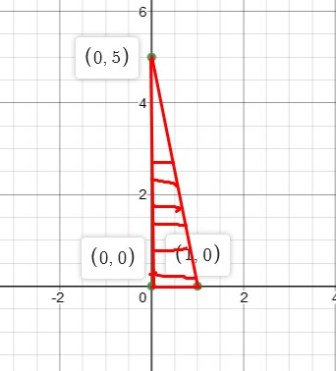# Evaluate the line integral. ? C 5 x y d x + 3 x 2 y d y C is the triangle with vertices (...

## Question:

Evaluate the line integral.

{eq}\oint_{C} 5xy \ dx + 3x^2 y \ dy {/eq}

{eq}C {/eq} is the triangle with vertices {eq}(0,0), (1,0), {/eq} and {eq}(0,5) {/eq}

## Evaluate the Integral:

Let {eq}C {/eq} be a positively oriented, piecewise smooth, simple, closed curve and let {eq}D {/eq} be the region enclosed by the curve. If {eq}P {/eq} and {eq}Q {/eq} have continuous first order partial derivatives on {eq}D {/eq} then Green's Theorem states that

{eq}\displaystyle \int_C P \ dx +Q \ dy = \int \int_R \left( \frac{\partial Q}{\partial x} - \frac{\partial P}{\partial y} \right) \ dA {/eq}

Solve this integral we get required solution

## Answer and Explanation:

Consider the integral

{eq}\displaystyle\oint_{C} 5xy \ dx + 3x^2 y \ dy {/eq}

C: is counterclockwise oriented triangle with vertices (0, 0), (1, 0), and (0,5)

Equation of line through the points (0,0) and (1,0) is {eq}\displaystyle y=0 {/eq}

Equation of line through the points (1,0) and (0,5) is {eq}\displaystyle y=-5x+5 {/eq}

Equation of line through the points (0,0) and (0,5) is {eq}\displaystyle x=0 {/eq}Solution by Green's method:

{eq}\displaystyle \oint_{C} 5xy \ dx + 3x^2 y \ dy = \int \int_R \left( \frac{\partial }{\partial x}( 3x^2 y) - \frac{\partial }{\partial y} (5xy)\right) \ dA\\ \displaystyle= \int \int_R (6xy-5x)\ dA\\ \displaystyle=\int_{x=0}^{1}\int_{y=0}^{-5x+5} (6xy-5x)\ dy\: dx\\ \displaystyle=\int_{x=0}^{1}\left ( 3xy^{2}-5xy \right )_{y=0}^{-5x+5}\: dx\\ \displaystyle\int _0^1\left(75x^3-125x^2+50x\right)dx\\ \displaystyle=\left ( \frac{75}{4}x^4-\frac{125}{3}x^3+25x^{2} \right )_{0}^{1}\\ \displaystyle=\frac{75}{4}-\frac{125}{3}+25\\ \displaystyle=\frac{25}{12} {/eq}

#### Learn more about this topic:Double Integrals & Evaluation by Iterated Integrals

from GRE Math: Study Guide & Test Prep

Chapter 15 / Lesson 4
401